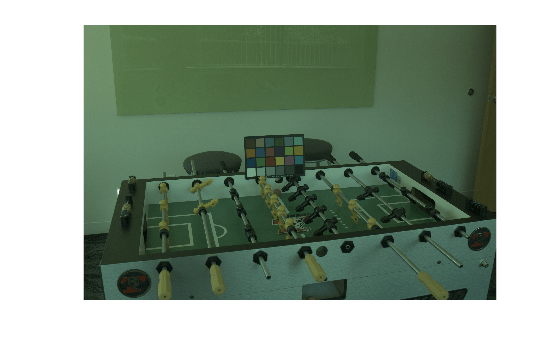# colorangle

Angle between two RGB vectors

## Syntax

``angle = colorangle(rgb1,rgb2)``

## Description

example

````angle = colorangle(rgb1,rgb2)` computes the angle in degrees between two RGB vectors.```

## Examples

collapse all

Read a test image. The image is the raw data captured with a Canon EOS 30D digital camera after correcting the black level and scaling the intensities to 16 bits per pixel. No demosaicing, white balancing, color enhancement, noise filtering, or gamma correction has been applied.

`RAW = imread("foosballraw.tiff");`

Interpolate using the `demosaic` function to obtain a color image. The color filter array pattern is RGGB.

`A = demosaic(RAW,"rggb");`

Display the image. Because the image is in linear RGB color space, apply gamma correction so the image appears correctly on the screen.

```A_sRGB = lin2rgb(A); imshow(A_sRGB)```The image contains a ColorChecker® chart. Specify the ground truth illuminant, which was calculated in advance using the neutral patches of the chart.

`illuminant_groundtruth = [0.0717 0.1472 0.0975];`

To avoid skewing the estimation of the illuminant, exclude the ColorChecker chart by creating a mask.

```mask = true(size(A,1), size(A,2)); mask(920:1330,1360:1900) = false;```

Run three different illuminant estimation algorithms: `illumwhite`, `illumgray`, and `illumpca`.

```illuminant_whitepatch = illumwhite(A,"Mask",mask); illuminant_grayworld = illumgray(A,"Mask",mask); illuminant_pca = illumpca(A,"Mask",mask);```

Compare each estimation against the ground truth by calculating the angle between each estimated illuminant and the ground truth using the `colorangle` function. The smaller the angle, the better the estimation. The magnitude of the estimation does not matter because only the direction of the illuminant is used to white-balance an image with chromatic adaptation.

`angle_whitepatch = colorangle(illuminant_whitepatch,illuminant_groundtruth)`
```angle_whitepatch = 5.0921 ```
`angle_grayworld = colorangle(illuminant_grayworld,illuminant_groundtruth)`
```angle_grayworld = 5.1036 ```
`angle_pca = colorangle(illuminant_pca,illuminant_groundtruth)`
```angle_pca = 5.0134 ```

The value of `angle_pca` is smallest, indicating that the PCA illuminant estimation algorithm is closest to the ground truth illumination for this image.

## Input Arguments

collapse all

First RGB vector, specified as a 3-element numeric vector.

Data Types: `single` | `double` | `uint8` | `uint16`

Second RGB vector, specified as a 3-element numeric vector.

Data Types: `single` | `double` | `uint8` | `uint16`

## Output Arguments

collapse all

Angle between RGB vectors, returned as a numeric scalar.

Data Types: `double`